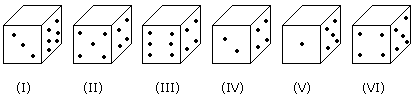# Verbal Reasoning - Dice

### Exercise :: Dice - Dice 2

Six dice with upper faces erased are as shows.The sum of the numbers of dots on the opposite face is 7.

1.

If even numbered dice have even number of dots on their top faces, then what would be the total number of dots on the top faces of their dice?

 A. 12 B. 14 C. 18 D. 24

Explanation:

Even numbered dice are: (II), (IV) and (VI)

No. of dots on the top face of (II) dice = 6

No. of dots on the top face of (IV) dice = 6

and No. of dots on the top face of (VI) dice = 6

Therefore Required total = 6 + 6 + 6 = 18

2.

If the odd numbered dice have even number of dots on their top faces, then what would be the total number of dots on the top faces of their dice?

 A. 8 B. 10 C. 12 D. 14

Explanation:

Odd numbered dice are : (II), (III) and (V)

No. of dots on the top faces of these dice are 2, 2 and 4 respectively.

Required total = 2 + 2 + 4 = 8

3.

If dice (I), (II) and (III) have even number of dots on their bottom faces and the dice (IV), (V) and (VI) have odd number of dots on their top faces, then what would be the difference in the total number of top faces between there two sets?

 A. 0 B. 2 C. 4 D. 6

Explanation:

No. of faces on the top faces of the dice (I), (II) and (III) are 5, 1 and 5 respectively.

Therefore, Total of these numbers = 5 + 1 + 5 = 11

No. of dots on the top faces of the dice (IV), (V) and (VI) are 1, 3 and 1 respectively.

Therefore, Total of these numbers = 1 + 3 + 1 = 5

Required difference = 11 - 5 = 6

4.

If the even numbers of dice have odd number of dots on their top faces and odd numbered dice have even of dots on their bottom faces, then what would be the total number of dots on their top faces?

 A. 12 B. 14 C. 16 D. 18

Explanation:

No. of dots on the top faces of the dice (II), (IV) and (VI) are 1, 1 and 1 respectively.

No. of dots on the top faces of the dice (I), (III) and (V) are 5, 5 and 3 respectively.

Required total = 5 + 5 + 3 + 1 + 1 + 1 = 16

5.

If the dice (I), (II) and (III) have even number of dots on their bottom faces, then what would be the total number of dots on their top faces?

 A. 7 B. 11 C. 12 D. 14